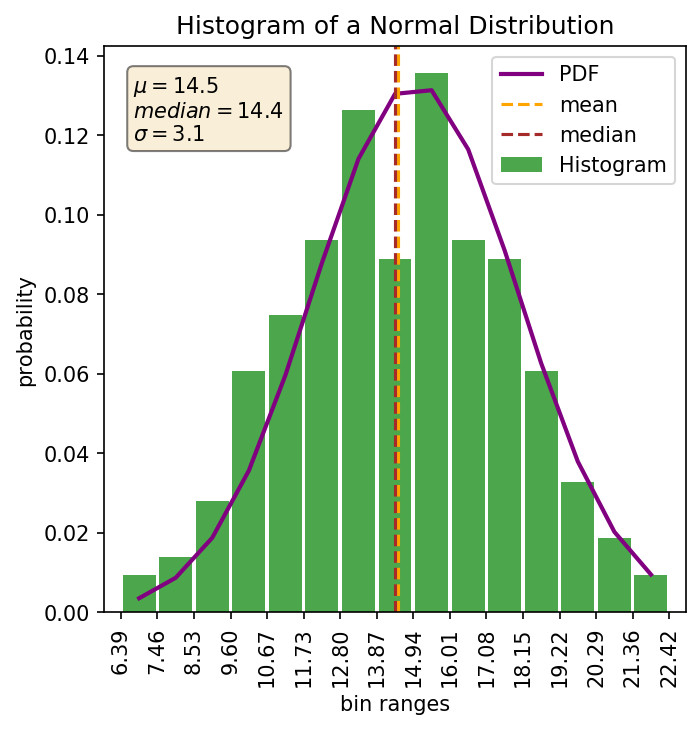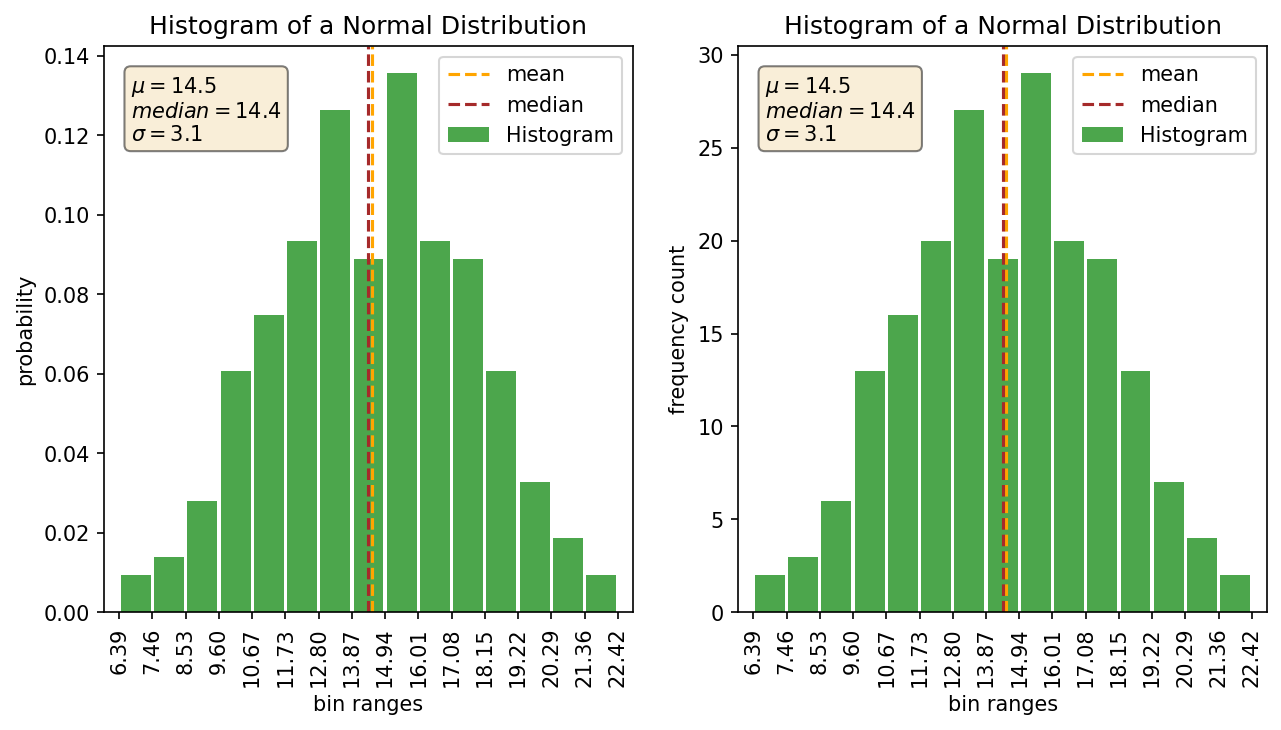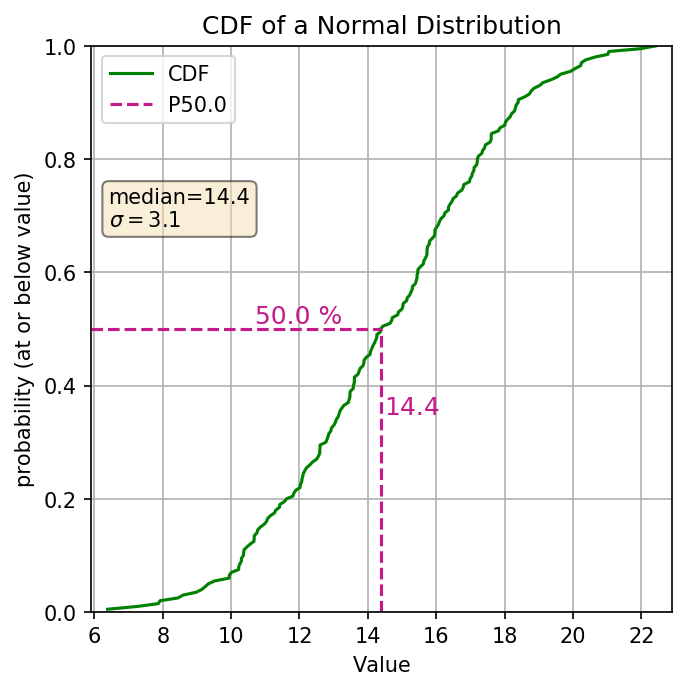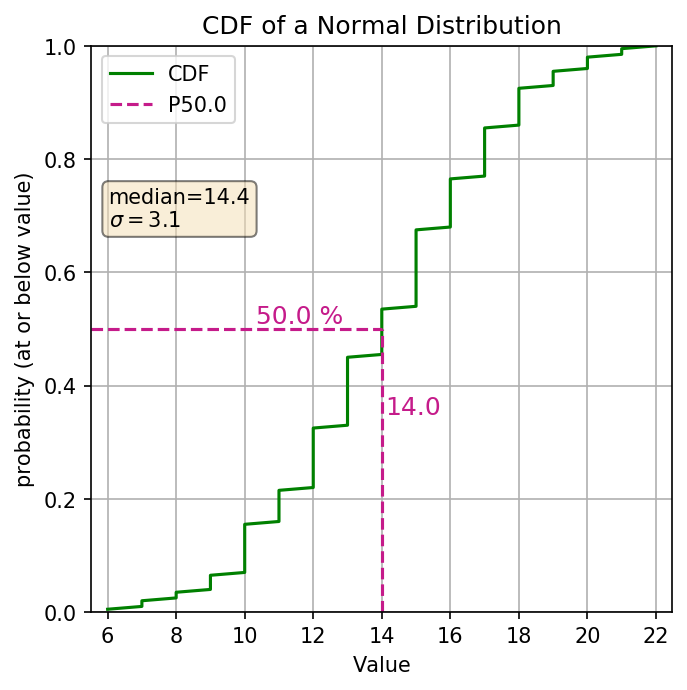# Histograms and CDF’s Part1: What are they?

This is the first part of a two part series on histograms and CDF’s (Cumulative Distribution Function). I find a lot of people new to data science, geostatistics, etc. are usually familiar and comfortable with histograms, however there is a lot to learn from CDF’s which is why I tend to rely on them more. This series will cover the following topics

1. What are they?
• Explain what a Histogram and CDF is
• Show what info we can easily read from each graph type
2. When should we use them?
• What are the pro’s and con’s of these graphical representations.

## TL:DR

At their heart, both the histogram and the CDF (Cumulative Distribution Function) are displaying similar information, but in different ways. A histogram can be thought of as an empirical estimation of the Probability Density Function (PDF) and represents the probability with areas. Technically the PDF would represent this with an “area under the curve”. Histograms are bar plots… so I guess we can say they represent this with the area of a bar! The CDF represents probability with vertical distances and is cumulative.

### Note: Example Data

The main plots for this post are all based on the same randomly generated distribution of numbers created using Numpy’s random number generator in Python. To keep it to something most people are familiar with, the distribution is based on a normal distribution. I’m using a small number of data so my distribution is not “perfect” but much more realistic. If you want to see how I do this you can check out my example notebook ex_histograms_and_cdfs which shows all of the plots used in this post.

## What’s a Histogram

Histograms are one commonly used graphical representation of a distribution of numbers. They are typically plotted in a bar graph style plot where the height of each bar shows the frequency of a bin and represents the probability that a number will fall within that bin. This is in essence a slice of the area under a curve. The width of the bar represents the bin size used to group the numbers (or the width of the slice). The total width of all bars shows the range of values in the distribution. Here’s an example showing the histogram and the estimated PDF for my normal distribution:As a quick side note: Many histogram plotting functions/programs out there by default plot a histogram with ‘Frequency’ on the y-axis. I don’t find this vary useful because the frequency doesn’t really mean anything without also knowing the how many samples are in your distribution. If you have a count of 30 samples in a bin and your sample size is 50 then that means something totally different than if you have a sample size of 10,000. Using the ‘Density’ option in numpy/matplotlib/plotly or whatever program you are using will convert it over to a probability value which I find way more useful. Here’s a quick example showing the density plot versus the frequency plot.## What’s a CDF

The cumulative distribution function (aka. CDF) is another graphical representation of the distribution of numbers (discrete, or continuous). The y-axis represents the cumulative probability, aka the percentile of your distribution. The x-axis is the values in your distribution (ordered from least to greatest). The line is using vertical distances to show the probabilities. Here’s an example of the same normal distribution.The CDF also works quite well for categorical distributions. Just be mindful of how the categories are related (ordinal or nominal). Here’s an example of the same normal distribution converted into a categorical distribution by converting all values to integers:## How do we read a Histograms

Ok so you probably already knew what a histogram was, and you might already know how to read a histogram, but to make sure we are on the same page lets look at what the histogram shows us. We’ll take the same normal distribution and plot it’s histogram, but this time in an interactive plot so you can look at some of the values yourself.